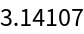#Function Repository Resource:

# BoolEval

Fast vectorized evaluation of array inequalities

Contributed by: Szabolcs Horvát
 ResourceFunction["BoolEval"][array>value] replaces elements of array which are greater than value with 1 and the rest with 0. ResourceFunction["BoolEval"][condition] takes a condition expressed in terms of >, ≥, <, ≤, ⩵, ≠ and logical operators, and evaluates it for each element of the arrays appearing in the condition, returning a Boolean array of 1s and 0s.

## Details and Options

ResourceFunction["BoolEval"] is intended for use with numerical arrays.
The arrays appearing in the conditions must have compatible sizes.
ResourceFunction["BoolEval"] has attribute HoldAll, and only processes operators that appear literally in the input.
The primary purpose of ResourceFunction["BoolEval"] is to achieve maximum performance, therefore it does not check its input for correctness. This is left to the programmer.
Effectively, ResourceFunction["BoolEval"][x>a] is equivalent to Boole@Thread[x>a].
Internally, ResourceFunction["BoolEval"] converts inequalities to expressions in terms of UnitStep and logical operators to arithmetic ones.
The BoolEval package contains the same function (BoolEval) as well as related utilities and documentation.

## Examples

### Basic Examples

Identify elements of an array that are larger than 5:

 In:=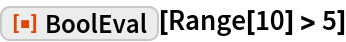Out=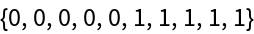Identify elements that are greater than or equal to 3 but less than 7:

 In:=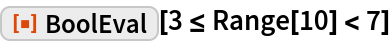Out=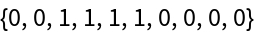### Scope

Higher dimensional arrays are supported:

 In:=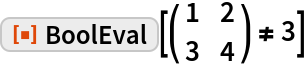Out=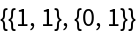Compare each row of a matrix with a different constant:

 In:=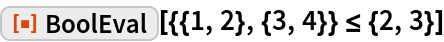Out=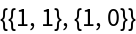Compare two arrays:

 In:=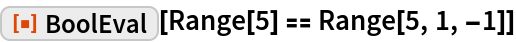Out=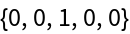BoolEval supports any expression that works with arithmetic and basic numerical operations:

 In:=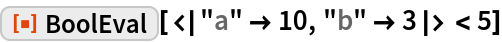Out=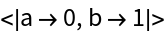BoolEval works with images:

 In:=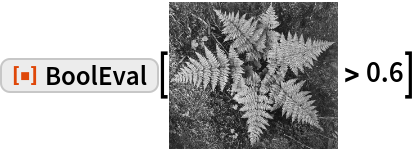Out=### Applications

Count the number of array elements that are greater than 0.3:

 In:=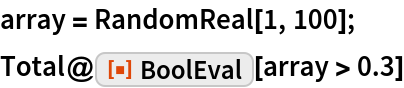Out=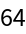Pick non-positive elements of an array:

 In:=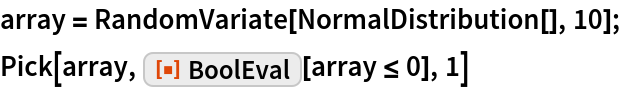Out=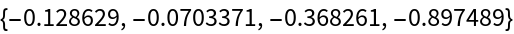Process an array much faster than it would be possible using Select or Cases:

 In:=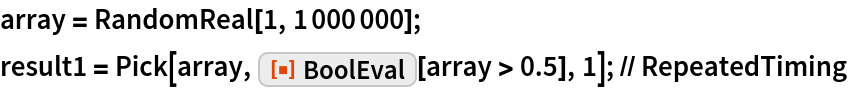Out=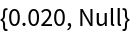In:=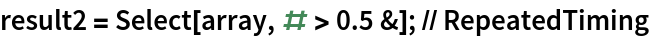Out=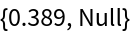In:=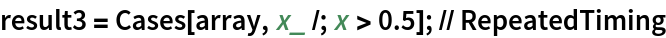Out=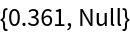In:=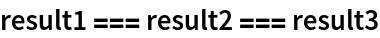Out=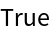### Properties and Relations

BoolEval effectively threads comparisons over arrays, then converts truth values to 0 or 1:

 In:=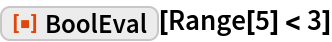Out=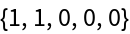In:=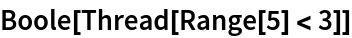Out=Literal truth values are also converted to 0s and 1s:

 In:=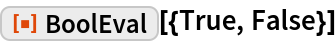Out=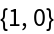Passing symbolic input to BoolEval reveals how it translates inequalities to UnitStep and logical operators to arithmetic ones:

 In:=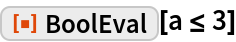Out=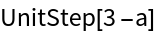In:=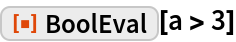Out=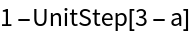In:=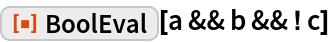Out=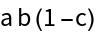### Possible Issues

Only those operators are processed which appear literally in the argument of BoolEval:

 In:=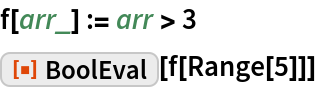Out=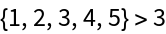In:=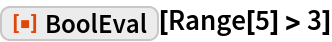Out=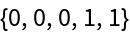Evaluate the argument explicitly using the Evaluate function to allow BoolEval to see the operators:

 In:=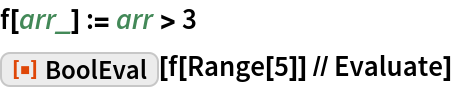Out=BoolEval is optimized for performance, and does not check its arguments for correctness:

 In:=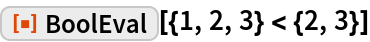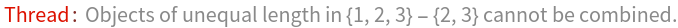Out=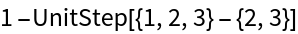The arrays in the argument must be numeric:

 In:=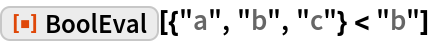Out=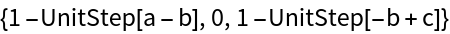### Neat Examples

Estimate the value of π using Monte Carlo integration:

 In:=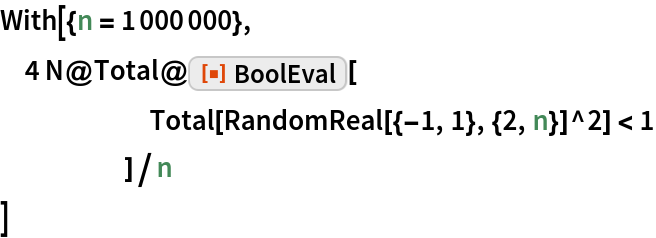Out=